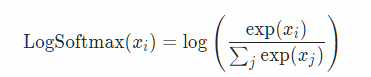# What is torch.nn really?

This topic has been created to discuss the idea, math and code behind Jeremy’s NN tutorial availalble at Pytorch website: https://pytorch.org/tutorials/beginner/nn_tutorial.html#

def log_softmax(x):
return x - x.exp().sum(-1).log().unsqueeze(-1)

def model(xb):
return log_softmax(xb @ weights + bias)

2. Also, I couldn’t understand the definition for negative loss-likelihood function:

def nll(input, target):
return -input[range(target.shape), target].mean()


Could you be more precise as to what you don’t understand ?

If you don’t understand the math of the function I’d suggest to go back to Jeremy’s lessons where he explains softmax.

If you don’t understand how’s the math is translated into code (because of the tensor operations maybe?) I’d suggest to set a sample x = torch.randn(...,...) to experiment with, and then apply the different functions successively to understand what they are doing. You can also look them up in PyTorch documentation.

Please let us know what you understand or if you still have questions, it will certainly help others tooI was reading that today too. That negative loss-likelihood is kinda weird, with the slicing [range(target.shape), target]. I should find the time to run the code. Will update if I figure what what it’s slicing

1 Like

Same here.

ok I think I got it. It’s using the target as column to get the logp for the correct target

1 Like

I think I found the answer to the first one. It’s all in the math.In the log_softmax(x) function, we are passing in xb * weights + bias. Then we are applying LogSoftMax.which is same as log(exp(x_i)) - log(\sum_j(exp(x_j))).

\log\exp{x_i} w.r.t e is x_i. So, we need to only calculate the second part, that is log\sum_j\exp{x_j}.

1 Like

And the fact that nll only takes the log-likelihood of the correct class allows us to use the target to slice out the correct column. Evil.

image taken from here

4 Likes

Hello

When I run the training loop in the “Neural net from scratch” part of the notebook, I print the loss after every batch. The loss is not getting smaller during the loop and also the accuracy ends up being 1. I dont get why this happens. Does this mean I get 100% training accuracy(overfitting) with just 1 neuron? And shouldn’t the loss be dropping during training?

Hello,

The loss should get smaller while running the training loop, as long as you did not change anything in the given kernel. However, it is pretty hard for the network to train with such a rough weights update policy : the model is kind of oscillating around the optimum you are trying to reach. That is why researchers have developed more sophisticated methods for weights updating.

Here, you have 10 neurons : one for each digit.

If you run the notebook, you get 100% accuracy after a couple of epochs. Actually, the kernel evaluate the accuracy on the last trained batch :

print(loss_func(model(xb), yb), accuracy(model(xb), yb))


print(loss_func(model(x_train), y_train), accuracy(model(x_train), y_train))